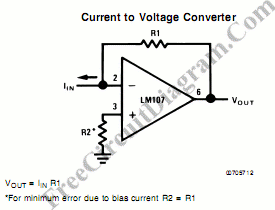# Current to Voltage ConverterIn the simplest form, current to voltage converter can be implemented as simple as one resistor,  since a voltage is developed when an electrical current flow against a resistance. The problem is that the resistance always affect the current by  decreasing the current flow, and the current to voltage conversion is not accurate. In some cases, the resistor that convert the current can be chosen as low as possible to make it much lower than the current source impedance, but it means a smaller conversion factor is also lowered, make the reading become more difficult.The above circuit will make the converter act like a zero impedance resistor, so any current from any source impedance would be perfectly converted. The feedback mechanism will keep the inverting input voltage to be same with the non-inverting one by adjusting its output, and this will make the inverting input virtually shorted to ground. [Source: National Semiconductor Application Notes]